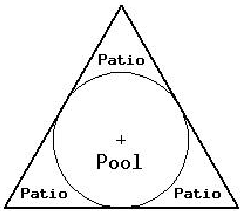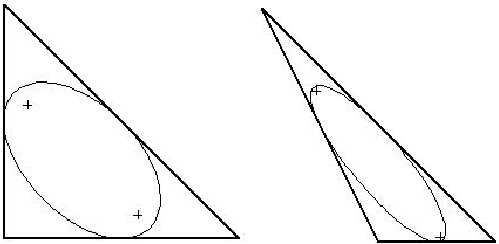Online JudgeProblem SetAuthorsOnline ContestsUser
Web Board
F.A.Qs
Statistical Charts
Problems
Submit Problem
Online Status
Prob.ID:
Register
Authors ranklist
Current Contest
Past Contests
Scheduled Contests
Award Contest
Register

Language:
Joe’s Triangular Gardens
 Time Limit: 1000MS Memory Limit: 65536K Total Submissions: 99 Accepted: 45

Description

Joe’s landscaping company specializes in gardens for computer geeks who have just had their company go public. One of his signature features is a round pool surrounded by a tiled patio in the form of an equilateral triangle where the edge of the pool is tangent to each side of the triangle at its midpoint.Unfortunately, some of Joe’s customers are not satisfied with an equilateral triangle, usually in the center of the garden. Some want it in a corner or next to a slope or some other layout. Joe would like the option of offering arbitrary triangular patios with an elliptical pool which is tangent to each side at the center of the side. For example:Joe knows how to draw an ellipse by putting two stakes in the ground (at the foci of the ellipse), tying a rope between them and dragging a marker stick inside the rope. What Joe would like is for the customer to determine where the corners of the triangle will be and then measure the location of the triangle vertices and compute where to put the stakes and how long to make the rope.

Write a program, which takes as input the three vertices (x1, y1), (x2, y2) and (x3, y3) of a triangle and computes an ellipse inscribed in the triangle, which is tangent to each side of the triangle at its midpoint. The output is the coordinates of the two foci of the ellipse and the length of the rope (which is the sum of the distances from the foci to any point on the ellipse.

Input

The first line of input contains a single integer N, (1 <= N <= 1000) which is the number of data sets that follow. Each data set consists of a single line of input containing 6 space separated floating point numbers x1 y1 x2 y2 x3 y3 giving the coordinates of the vertices of a triangle.

Output

For each data set, you should generate one line of output with the following values: The data set number as a decimal integer (start counting at one), a space and five floating point values accurate to two decimal places each separated by a single space. The values are fx1 fy1 fx2 fy2 rl where (fx1, fy1) is one focus of the ellipse, (fx2, fy2) is the other focus of the ellipse and rl is the sum of the distances from the foci to any point on the ellipse (e.g. the length of the rope). The foci should be listed in increasing lexicographical order (i.e. fx1 <= fx2 and if fx1 = fx2, fy1 <= fy2). Note that in the case the ellipse is a circle, the two foci are the same (e.g. the center of the circle).

Sample Input

```3
100 100 200 273.2051 300 100
100 100 100 300 300 100
100 200 100 300 300 100```

Sample Output

```1 200.00 157.71 200.00 157.76 115.47
2 119.53 213.81 213.81 119.53 163.30
3 103.94 253.14 229.40 146.86 170.51```

Source

[Submit]   [Go Back]   [Status]   [Discuss]Home PageGo BackTo top